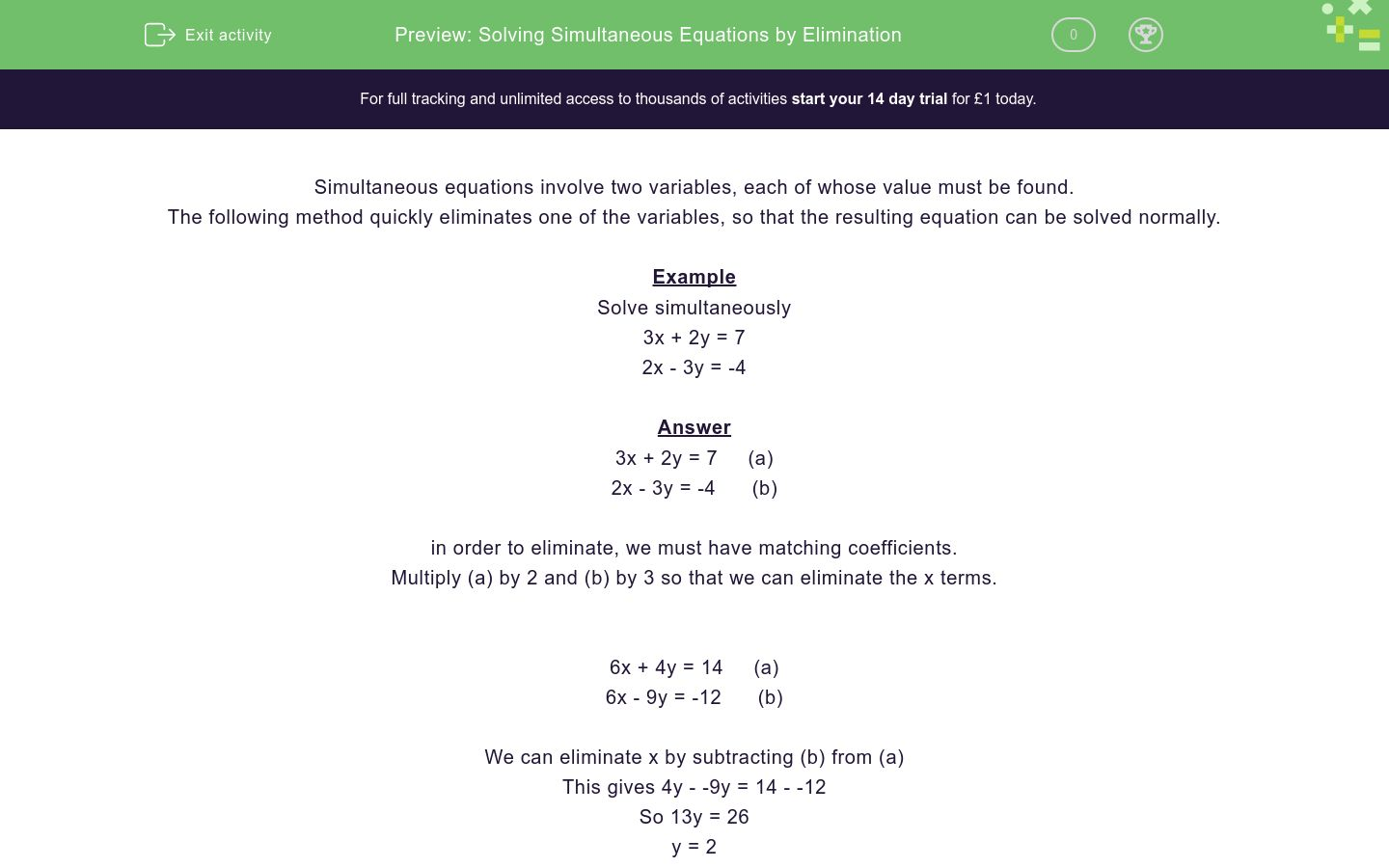# Solving Simultaneous Equations by Elimination

In this worksheet, students solve simultaneous equations by eliminating one variable.Key stage:  KS 4

Curriculum topic:  Algebra

Curriculum subtopic:  Solve Simultaneous Equations in 2 Variables

Difficulty level:### QUESTION 1 of 10

Simultaneous equations involve two variables, each of whose value must be found.

The following method quickly eliminates one of the variables, so that the resulting equation can be solved normally.

Example

Solve simultaneously

3x + 2y = 7

2x - 3y = -4

3x + 2y = 7     (a)

2x - 3y = -4      (b)

in order to eliminate, we must have matching coefficients.

Multiply (a) by 2 and (b) by 3 so that we can eliminate the x terms.

6x + 4y = 14     (a)

6x - 9y = -12      (b)

We can eliminate x by subtracting (b) from (a)

This gives 4y - -9y = 14 - -12

So 13y = 26

y = 2

Substitute this y value into one of the equations, say (a) to get

3x + 4 = 7

3x = 3

x = 1

Check by putting this into the other equation (b) to get 2 x 1 - 3 x 2 = 2 - 6 = -4

Solution is x = 1, y = 2

Solve simultaneously:

3x + 2y = 13

5x - 3y = 9

x = 4, y = 1

x = 12, y = 3

x = 3, y = 2

Solve simultaneously:

3x + 2y = 14

13x - 5y = 6

x = 4, y = 2

x = 2, y = 4

x = 6, y = -2

Solve simultaneously:

4x + 3y = 15

5x - 4y = 11

x = 2, y = 1

x = 3, y = 1

x = 4, y = 2

Solve simultaneously:

2x + 5y = 31

7x - 2y = 11

x = 3, y = 5

x = 13, y = 1

x = 4, y = -2

Solve simultaneously:

7x + 2y = 20

8x - 5y = 1

x = 6, y = -11

x = 5, y = 5

x = 2, y = 3

Solve simultaneously:

5x + 6y = 17

3x + 5y = 13

x = 2, y = 4

x = 3, y = 4

x = 1, y = 2

Solve simultaneously:

4x + 3y = 22

3x - 4y = 4

x = 4, y = 2

x = 2, y = 3

x = 10, y = -6

Solve simultaneously:

3x + 7y = 0

2x - 5y = 29

x = 3, y = 7

x = 7, y = -3

x = 21, y = 1

Solve simultaneously:

5x + 3y = -33

3x + 2y = -20

x = 1, y = 3

x = 1, y = 4

x = -6, y = -1

Solve simultaneously:

6x + 5y = 29

3x + 2y = 11

x = 1½, y = 4

x = -1, y = 7

x = 1, y = 10

• Question 1

Solve simultaneously:

3x + 2y = 13

5x - 3y = 9

x = 3, y = 2
• Question 2

Solve simultaneously:

3x + 2y = 14

13x - 5y = 6

x = 2, y = 4
• Question 3

Solve simultaneously:

4x + 3y = 15

5x - 4y = 11

x = 3, y = 1
• Question 4

Solve simultaneously:

2x + 5y = 31

7x - 2y = 11

x = 3, y = 5
• Question 5

Solve simultaneously:

7x + 2y = 20

8x - 5y = 1

x = 2, y = 3
• Question 6

Solve simultaneously:

5x + 6y = 17

3x + 5y = 13

x = 1, y = 2
• Question 7

Solve simultaneously:

4x + 3y = 22

3x - 4y = 4

x = 4, y = 2
• Question 8

Solve simultaneously:

3x + 7y = 0

2x - 5y = 29

x = 7, y = -3
• Question 9

Solve simultaneously:

5x + 3y = -33

3x + 2y = -20

x = -6, y = -1
• Question 10

Solve simultaneously:

6x + 5y = 29

3x + 2y = 11